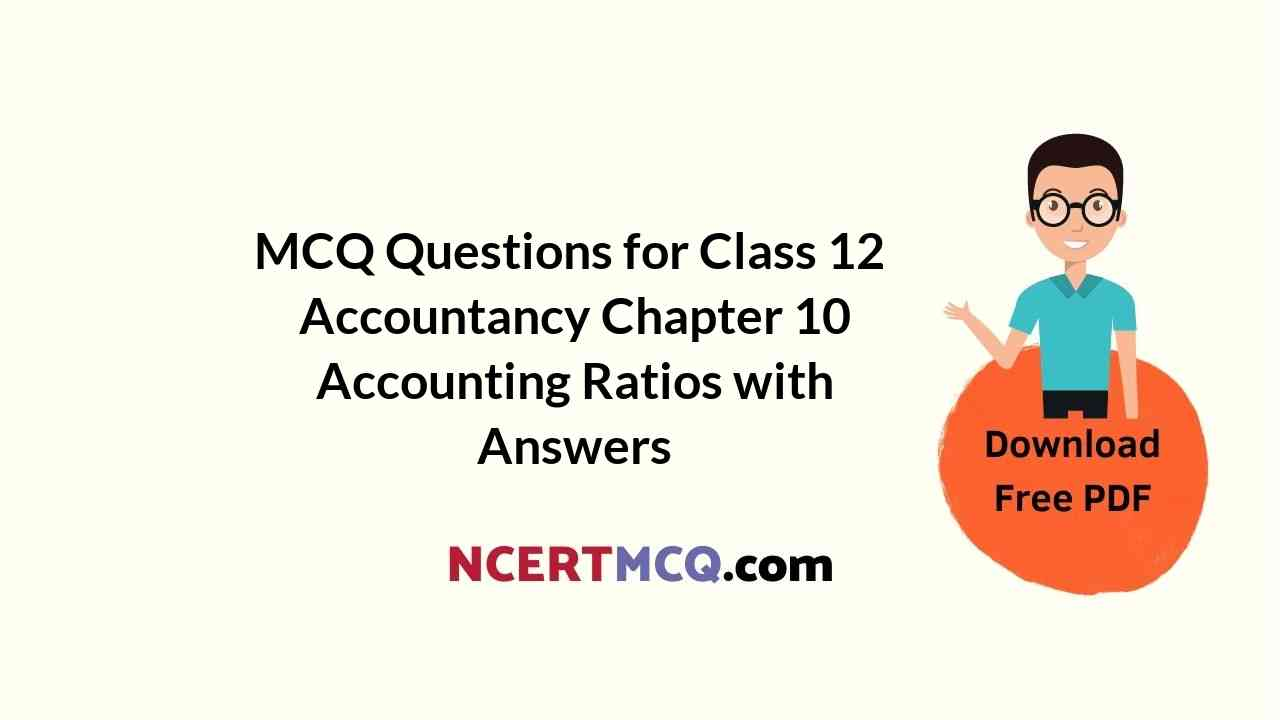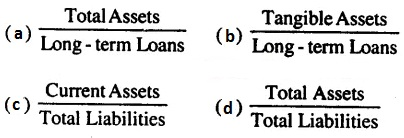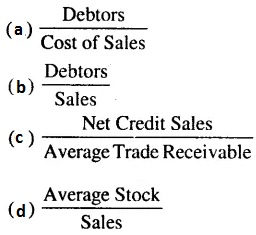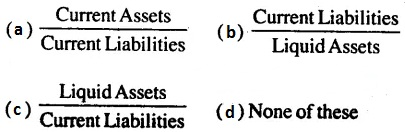Check the below NCERT MCQ Questions for Class 12 Accountancy Chapter 10 Accounting Ratios with Answers Pdf free download. MCQ Questions for Class 12 Accountancy with Answers were prepared based on the latest exam pattern. We have provided Accounting Ratios Class 12 Accountancy MCQs Questions with Answers to help students understand the concept very well.

## Accounting Ratios Class 12 MCQs Questions with Answers

Accounting Ratios Class 12 MCQ Question 1.
The formula for ascertaining Total Assets to Debt Ratio is:Accounting Ratios MCQ Question 2.
Proprietory Ratio indicates the relationship between proprietor’s funds and….
(a) Reserve
(b) Share Capital
(c) Total Assets
(d) Debentures

MCQ Of Accounting Ratios Class 12 Question 3.
Proprietory ratio is calculated by the following formula:Accounting Ratios MCQs Class 12 Question 4.
Which one of the following ratios is most important in determining the long-term solvency of a company ?
(a) Profitability Ratio
(b) Debt-Equity Ratio
(c) Stock Turnover Ratio
(d) Current RatioAccounting Ratios MCQs Question 5.
Total Assets ₹ 8,10,000
Total Liabilities ₹ 2,60,000
Current Liabilities ₹ 40,000
Debt-equity ratio is:
(a) 0.05 : 1
(b) 0.4 : 1
(c) 2.5 : 1
(d) 4 : 1

MCQ On Ratio Analysis Class 12 Question 6.
Equity share capital ₹ 15,00,000
Reserve and Surplus ₹ 7,50,000
Total Assets ₹ 45,00,000
Properletory Ratio ?
(a) 50%
(b) 33.3%
(c) 200%
(d) 60%

Ratio Analysis MCQ Class 12 Question 7.
Total Assets ₹ 7,70,000
Total Liabilities ₹ 2,60,000
Current Liabilities ₹ 40,000
Total Assets to Debt Ratio is:
(a) 3.5 : 1
(b) 2.56 : 1
(c) 2.8 : 1
(d) 3 : 1

MCQ On Accounting Ratios Question 8.
Profitability Ratios are generally expressed in :
(a) Simple Ratio
(b) Percentage
(c) Times
(d) None of these

Ratio Analysis Class 12 MCQ Question 9.
The ratios are primarily measures of earning capacity of the business.
(a) Liquidity
(b) Activity
(c) Debt
(d) Profitability

Question 10.
The gross profit ratio is the ratio of gross profit to :
(a) Net Cash Sales
(b) Net Credit Sales
(c) Closing Stock
(d) Net Total Sales

Question 11.
Operating Ratio is:
(a) Profitability Ratio
(b) Activity Ratio
(c) Solvency Ratio
(d) None of these

Question 12.
Which of the following is an operating’ income ?
(a) Sale of Merchandise
(b) Interest Income
(c) Dividend Income
(d) Profit on the sale of old car

Question 13.
Which of the following non-operating expense?
(a) Rent
(b) Selling Expenses
(c) Wages
(d) Loss on Sale of Machinery

Answer: (d) Loss on Sale of Machinery

Question 14.
The following groups of ratios primarily measure risk
(a) Liquidity, activity and profitability
(b) Liquidity, activity and common stock
(c) Liquidity, activity and debt
(d) Activity, debt and profitability

Answer: (d) Activity, debt and profitability

Question 15.
To know the return on investment, by capital employed we mean:
(a) Net Fixed Assets
(b) Current Asset-Current Liabilities
(c) Gross Block
(d) Fixed Assets + Current Assets-Current Liabilities

Answer: (d) Fixed Assets + Current Assets-Current Liabilities

Question 16.
The term fixed assets include :
(a) Cash
(b) Machinery
(c) Debtors
(d) Prepaid Expenses

Question 17.
Ratio based on figures of profit & loss as well a the Balance sheet are:
(a) Profitability Ratios
(b) Operation Ratio
(c) Liquidity Ratio
(d) Composite Ratio

Question 18.
Debtors Turnover Ratio :Question 19.
When opening stock is ₹ 50,000 closing stock ₹ 60,000 and cost of goods sold is ₹ 2,20,000, then stock turn over ratio is:
(a) 2 times
(b) 3 times
(c) 4 times
(d) 5 times

Question 20.
What does Creditors Turnover Ratio take into account:
(a) Total credit purchases
(b) Total credit sales
(c) Total cash sales
(d) Total cash purchases

Question 21.
Cost of goods sold :
(a) Sales – Net profit
(b) Sales – Gross profit
(c) Purchases – Opening Stock
(d) None of the above

Answer: (b) Sales – Gross profit

Question 22.
The ideal liquid ratio is :
(a) 2 : 1
(b) 1 : 1
(c) 5 : 1
(d) 4 : 1

Question 23.
The ideal current ratio is :
(a) 2 : 1
(b) 1 : 2
(c) 3 : 2
(d) 3 : 4

Question 24.
Operating Ratio is:
(a) Profitability Ratio
(b) Activity Ratio
(c) Solvency Ratio
(d) None of these

Question 25.
Profitability Ratio is generally shown in :
(a) Simple Ratio
(b) Percentage
(c) Times
(d) None of these

Question 26.
If sales is 7 4,20,000 sales returns is 7 20,000 and cost of goods sold 7 3,20,000 gross profit ratio will be :
(a) 20%
(b) 25%
(c) 15%
(d) 10%

Question 27.
Stock turnover ratio comes under :
(a) Liquidity Ratio
(b) Profitability Ratio
(c) Activity Ratio
(d) None of these

Question 28.
The satisfactory ratio between internal and external equity is. :
(a) 1 : 2
(b) 2 : 1
(c) 3 : 1
(d) 4 : 1

Question 29.
Current Ratio includes:
(a) Stock
(b) Debtors
(c) Cash
(d) All of these

Question 30.
Current Ratio =
(a) Current Assets/Current Liabilities
(b) Liquid Assets/Current Liabilities
(c) Liquid Assets/Current Assets
(d) Fixed Assets/Current Assets

Question 31.
Liquid Assets include :
(a) Bills Receivable
(b) Debtors
(c) Cash Balance
(d) All of theseQuestion 32.
Which of the following assets is not taken into consideration in calculating acid-test ratio ?
(a) Cash
(b) Bills Receivable
(c) Stock
(d) None of these

Question 33.
When Cash is 7 10,000 Stock is 7 25,000, B/R is 7 5,000 Creditors is 7 22,000 and Bank Overdraft is 7 8,000 then current ratio is :
(a) 2 : 1
(b) 4 : 3
(c) 3 : 4
(d) 1 : 2

Question 34.
The two basic measures of liquidity are :
(a) Inventory Turnover and Current Ratio
(b) Current Ratio and Liquid Ratio
(c) Current Ratio and Average Collection Period
(d) Current Ratio and Debtors Turnover Ratio

Answer: (b) Current Ratio and Liquid Ratio

Question 35.
Liquidity Ratio:Question 36.
The term ‘Current Liabilities’ does not include: .
(a) Sundry Creditors
(b) Debentures
(c) Bills Payable
(d) Outstanding Expenses

Question 37.
The term‘Current Assets’include
(a) Long-term Investment
(b) Short-term Investment
(c) Furniture
(d) Preliminary Expenses

Question 38.
Liquid Ratio is also known as:
(a) Current Ratio
(b) Quick Ratio
(c) Capital Ratio
(d) None of these

Question 39.
To test the liquidity of a concern which of the following ratios is useful ?
(a) Capital Turnover Ratio
(b) Acid Test Ratio
(c) Stock Turnover Ratio
(d) Net Profit Ratio

Question 40.
Which of the following transactions will improve the current ratio ?
(a) Purchase of good for cash
(c) Payment of creditors
(d) Credit purchase of goods

Question 41.
Debt-equity ratio is :
(a) Liquidity Ratio
(b) Activity Ratio
(c) Solvency Ratio
(d) Operating Ratio

Question 42.
The formula for finding out Debt-Equity Ratio is:
(a) Long-term Debts/Shareholders’ Funds
(b) Debentures/Equity Capital
(c) Net Profit/Total Capital
(d) None of these